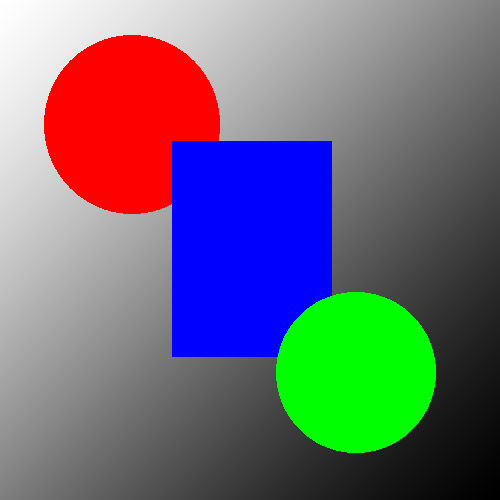# Convert an Image to Gray Scale in C#Sometimes you need to take a color image and remove all of the color from it leaving just shades of gray. There are 3 common ways of performing this calculation, each yielding slightly different results.

• The Lightness method
• The Average method
• The Luminosity method

They are all very easy to implement in C#. In this example we’ll use the Luminosity Method since that is the default method used in most image manipulation programs.

To convert an image to gray scale we need to look at each pixel, calculate the luminosity for that pixel, and set that pixels value so that each red, green, and blue are equal to the luminosity. The formula for luminosity is:

Luminosity = 0.21 R + 0.72 G + 0.07 B

In C# we need to store the luminosity as an integer, but the compiler is going to calculate it as a double, so we’re going to need to convert it to an int. We’re using Convert.ToInt for the conversion, but you could use an (int) cast instead if you understand the difference.

Here’s the code to load an image, convert it to gray scale, and save the results as a PNG.

``````// convert an image to gray scale using the luminosity method
private static void ToGrayscaleLuminosity(Bitmap bmp)
{
var lockedBitmap = new LockBitmap(bmp);
lockedBitmap.LockBits();

for (int y = 0; y < lockedBitmap.Height; y++)
{
for (int x = 0; x < lockedBitmap.Width; x++)
{
var oldColor = lockedBitmap.GetPixel(x, y);
// this is called a "Luminosity Average" method because it takes
// a weigted average of R, G, and B
var luminosity = ((0.21* oldColor.R) + (0.72 * oldColor.G) + (0.07 * oldColor.B))/3;
var c = Convert.ToInt32(luminosity);
var newColor = Color.FromArgb(255, c, c, c);
lockedBitmap.SetPixel(x, y, newColor);
}
}
lockedBitmap.UnlockBits();
}``````

#### Results of Convert to Gray Scale

Here are the results of converting to gray scale using luminosity.

##### Source Image##### Output ImageYou can see that the blue was turned to almost black. This is because blue has a very low coefficient in the luminosity equation.

#### Alternate Formula for Gray Scale

If you do not like the results of this conversion you can always change the luminosity formula to be a straight average. To do that replace this line:

``var luminosity = ((0.21* oldColor.R) + (0.72 * oldColor.G) + (0.07 * oldColor.B))/3;``

With this:

``var luminosity = (oldColor.R + oldColor.G + oldColor.B)/3;``

This is called an “Average” formula because it takes the simple mathematical average of red, green, and blue and uses that value for the new components.

#### Other Image Processing Guides

If you like this guide you might want to check out some of our other C# Image Processing Guides.

This site uses Akismet to reduce spam. Learn how your comment data is processed.